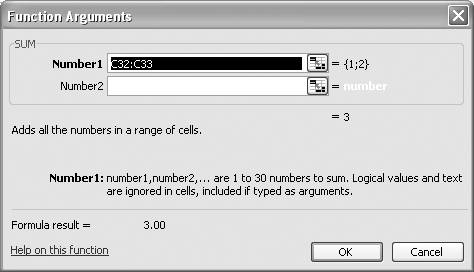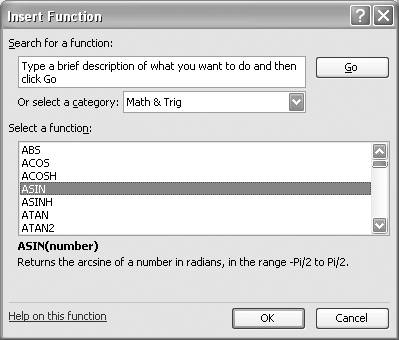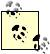# Exploring Functions

Problem

The basic mathematical operators (+, -, /, *, and ^) are not enough to perform all the calculations you need.

Solution

Use Excel's built-in functions, such as ABS( ), SQRT( ), and SIN( ), as needed.

Discussion

Throughout this book, I'm going to use all sorts of built-in functions in various calculations from data analysis to unit conversions to various engineering calculations. In this recipe I want to make you aware of the wide variety of built-in functions and how to access them in your spreadsheets.

Excel has many built-in functions, which can be organized in the following categories:

Database functions

Database functions include functions that allow you to get information from database entries and perform some statistical analyses of data contained in databases.

Date and time functions

Date and time functions include functions that allow you work with and perform calculations using dates and times. For example, you may want to calculate the number of working days between two dates, in which case you can use the NETWORKDAYS function. There are many others, including functions to get the current date and time.

Engineering functions

Engineering functions include functions that allow you to work with complex numbers, convert between number systems, and convert between systems of measurement. There are also many other specialized functions for working with Bessel and Delta functions, among others.

Financial functions

Financial functions include many functions that help you analyze things like accrued interest, depreciation, annuities, and investments, among many others.

Logical functions

Logical functions include a small set of useful logical functions . For example, the IF function allows you to evaluate a logical expression and return one value if the expression is true and another value if it is false. There are other functions including AND, OR, and NOT, among others, that help you construct logical expressions.

Lookup functions

Lookup functions are useful for looking up data in tables, matching values, returning rows or columns for cell references, and other tasks.

Math functions

Math functions help you perform calculations, and include operations such as taking the absolute value of a number, finding square roots, working with logarithms and exponential functions, summing values of data contained in rows or columns, and working with trigonometric functions. This is perhaps the most used set of functions for the sorts of calculations discussed in this book.

Statistical Functions

Statistical functions are useful for calculating averages, standard deviations, variances, among many other calculations. There is also a set of functions useful for working with probability and distributions, including binomial, Weibull, and normal distributions, among others.

Text and data functions

Text and data functions include a set of functions for manipulating text and converting numeric data to text. For example, there are functions to compare text strings, search for a substring within a larger text string, convert a string to all upper- or lowercase, and replace characters within a string.

There are a few other categories of built-in functions that I didn't summarize here. These functions are rather specialized and are useful for working with external programs and databases and collecting information from the host operating system, among other tasks.

The recipes throughout the remainder of this book will use many built-in functions, most notably, but not limited to, the math, statistical, engineering, and logical functions. I'm not going to list all of the available functions here, since they are already documented fairly well within Excel itself and there are also many books that serve as complete Excel function references. I do want to show you how to access these built-in functions now so you can be familiar with the process when you read the more specific recipes to follow in later chapters of this book.

The easiest way to use a function in a formula is to simply type the function. For example, to sum the values contained in a column consisting of the cell range C1:C10, you can write a formula using the SUM function like this: =SUM(C1:C10). In this case, SUM is the function and the cell range is the argument of the function.

When typing such a formula in a cell, as soon as you type the opening parenthesis after the function name, Excel will display a small, helpful information box below your formula, showing what arguments are expected (assuming you've typed a valid built-in function and spelled it correctly). At this point, you can type the arguments directly or, if the argument calls for a cell range, you can actually point and click to select the cell range, and Excel will automatically complete the cell reference argument for you. This reduces working with cell references in formulas to a point-and-click operation rather than typing everything out manually.

When typing a function, if you'd like more help on the function aside from the argument list that's automatically displayed, you can press the fx icon (called the Insert Function button ) in the formula bar to display the Function Arguments dialog box (see Figure 1-14).

Figure 1-14. Function Arguments dialog boxThe Function Arguments dialog box displays controls and notes to help you construct the arguments for the function. There's also a link to more help on the specific function, as shown in the lower-left corner of the dialog box in Figure 1-14.

Of course, the method of using functions discussed so far requires that you at least know the function name that you'd like to use. If you don't know or can't remember the name, you can either look it up in Excel Help or you can use the formula bar and the Insert Function dialog box to help you find appropriate functions.

To use the Insert Function dialog, select the formula bar to begin writing a formula and then press the fx icon when you need to look up a function. This will bring up the Insert Function dialog box (see Figure 1-15).

Figure 1-15. Insert Function dialog boxWith the Insert Function dialog box open, you may type a short description of what you're looking for in the "Search for a function" field. Or you can select a category from the drop-down list to view a list of functions within that category. From there you can select the specific function you need and press OK. When you press OK, the Function Argument dialog box will open to assist you with supplying function arguments. When you press OK in the Function Argument dialog box, you'll see your selected function, including arguments, appear in the formula bar; at this point, you can continue writing your formula.You can nest functions. For example, you can use a formula like =SQRT(ABS(C5)) to return the square root of the absolute value of the value contained in cell C5.

Excel Help contains a handy function reference. I usually have the task pane opened up with the Excel Help page displayed and open to the function reference section of the help guide. To open the function reference, open the task pane (Ctrl-F1) and select Excel Help from the drop-down list at the top of the pane. Click the "Table of Contents" link, look for the topic "Working with Data," and click it. Now look for the subtopic "Function Reference" and click it to see the various function categories, which you can click to reveal specific topics on each built-in function.

There are also many useful Excel function reference books on the market, such as Excel 2000 in a Nutshell (O'Reilly), by Jinjer Simon. It contains a concise reference to all of the menu options and built-in functions in Excel.Excel Scientific and Engineering Cookbook (Cookbooks (OReilly))
ISBN: 0596008791
EAN: 2147483647
Year: N/A
Pages: 206
Authors: David M Bourg